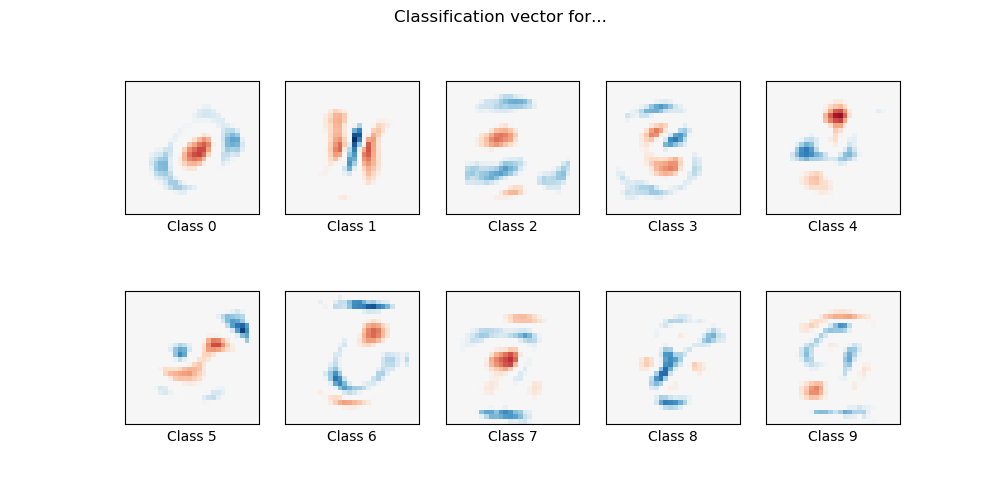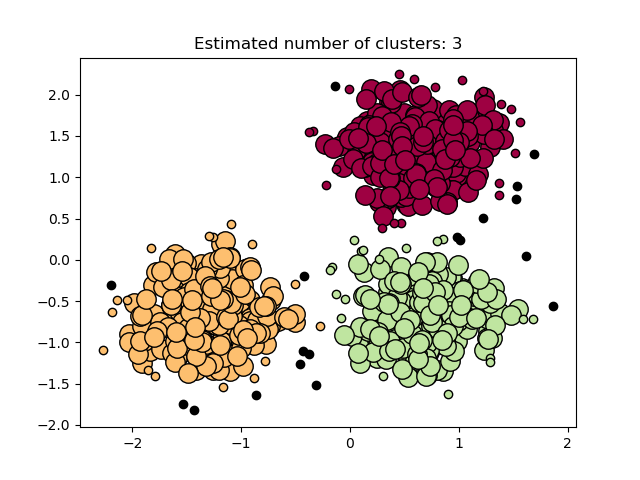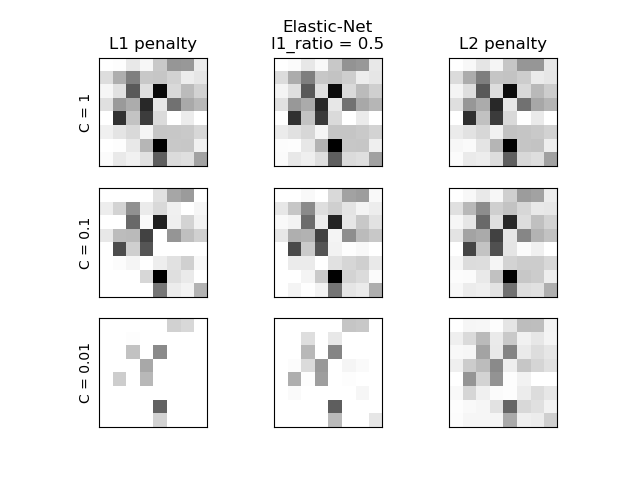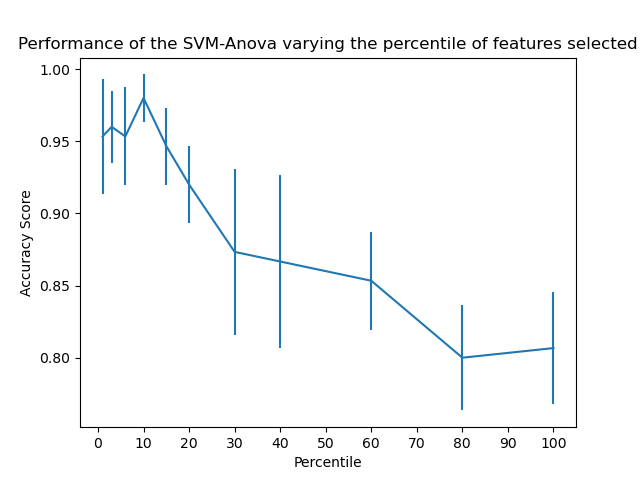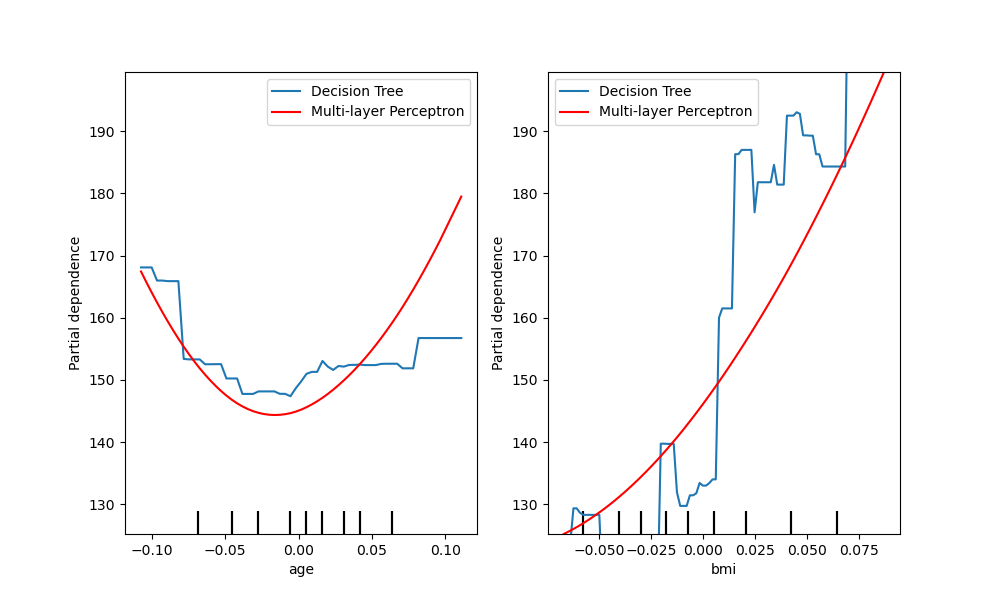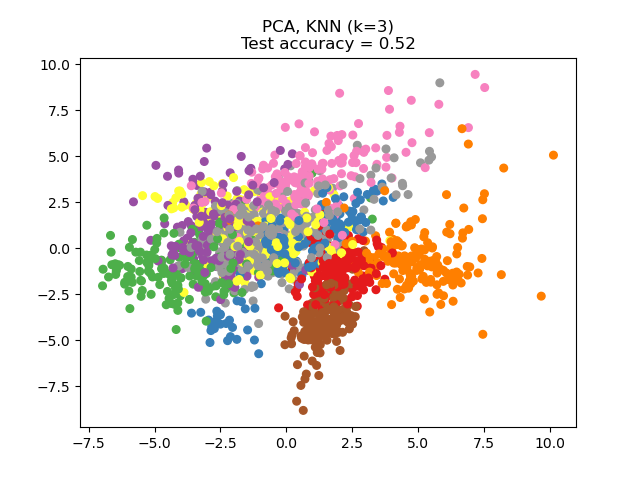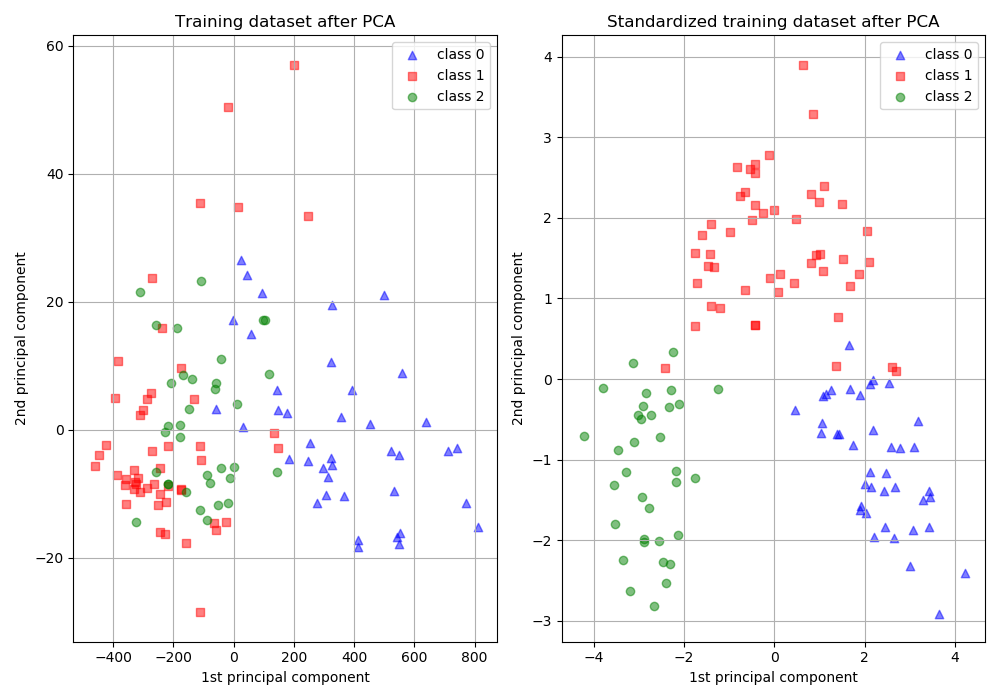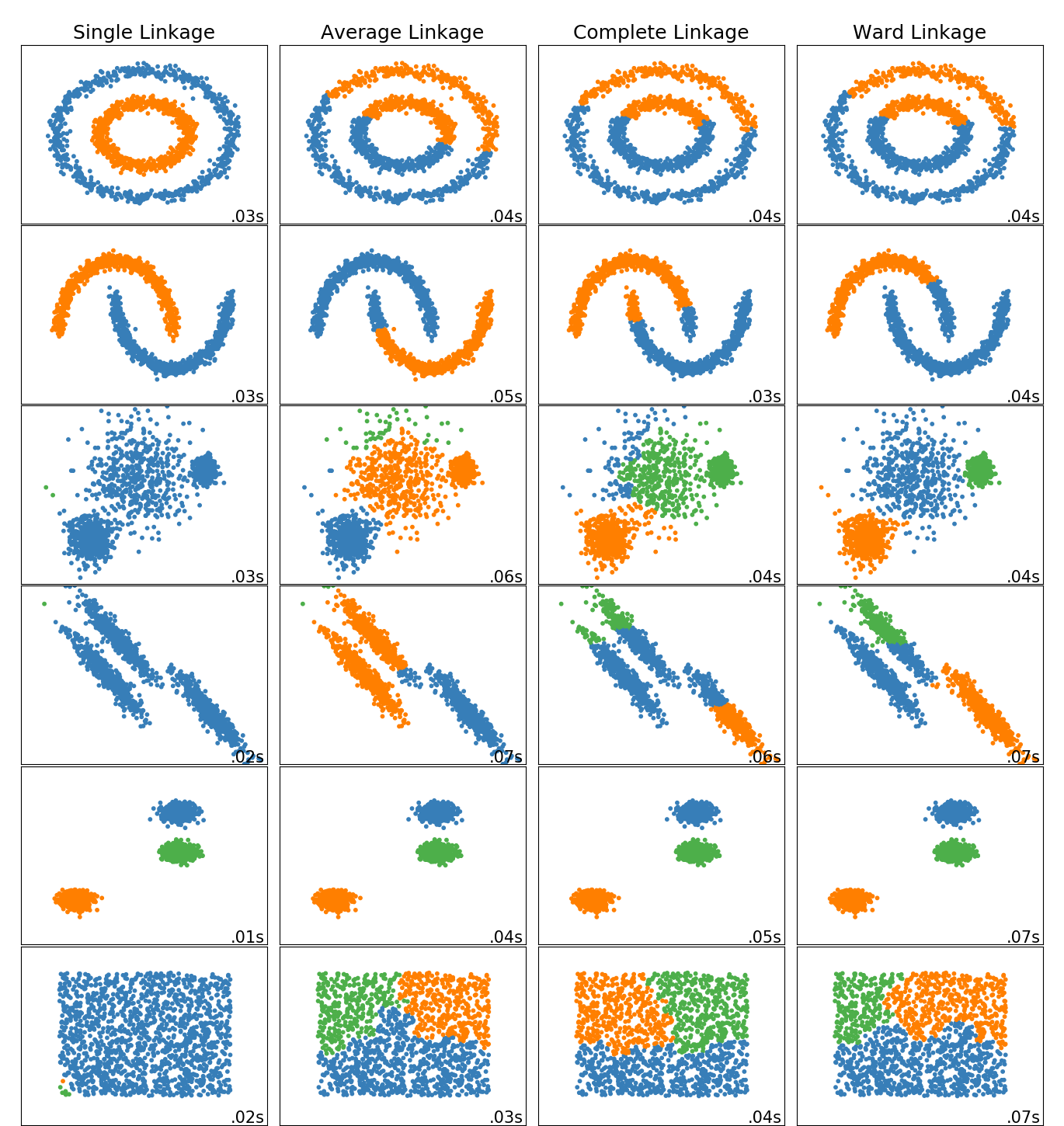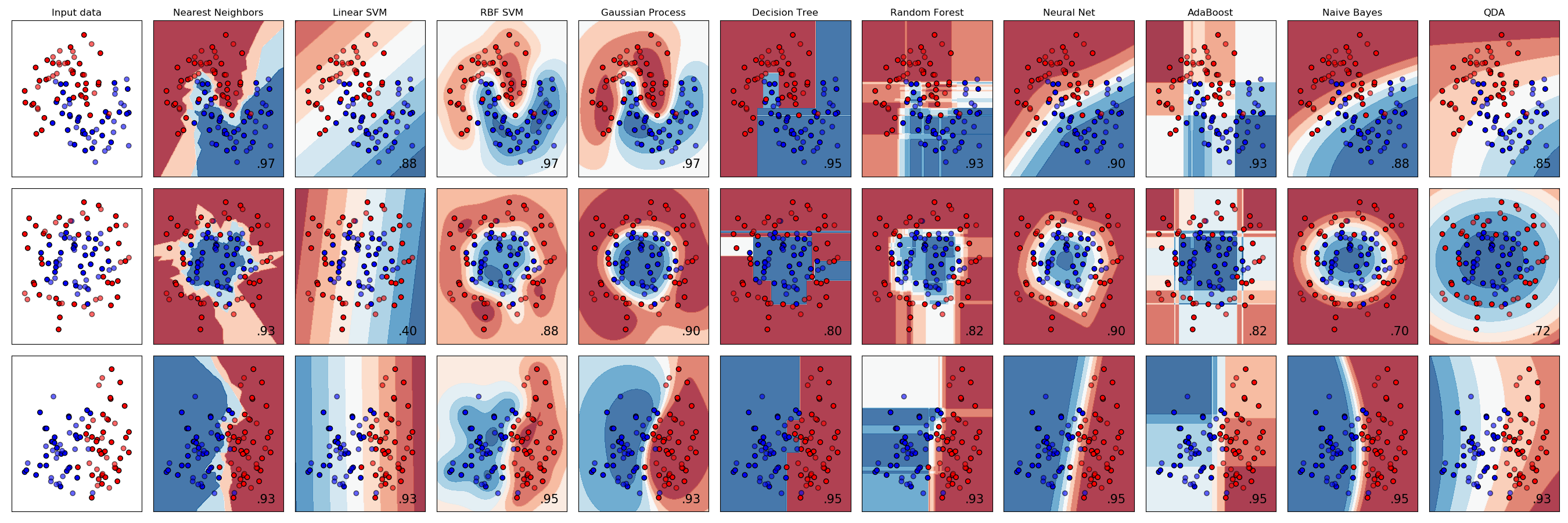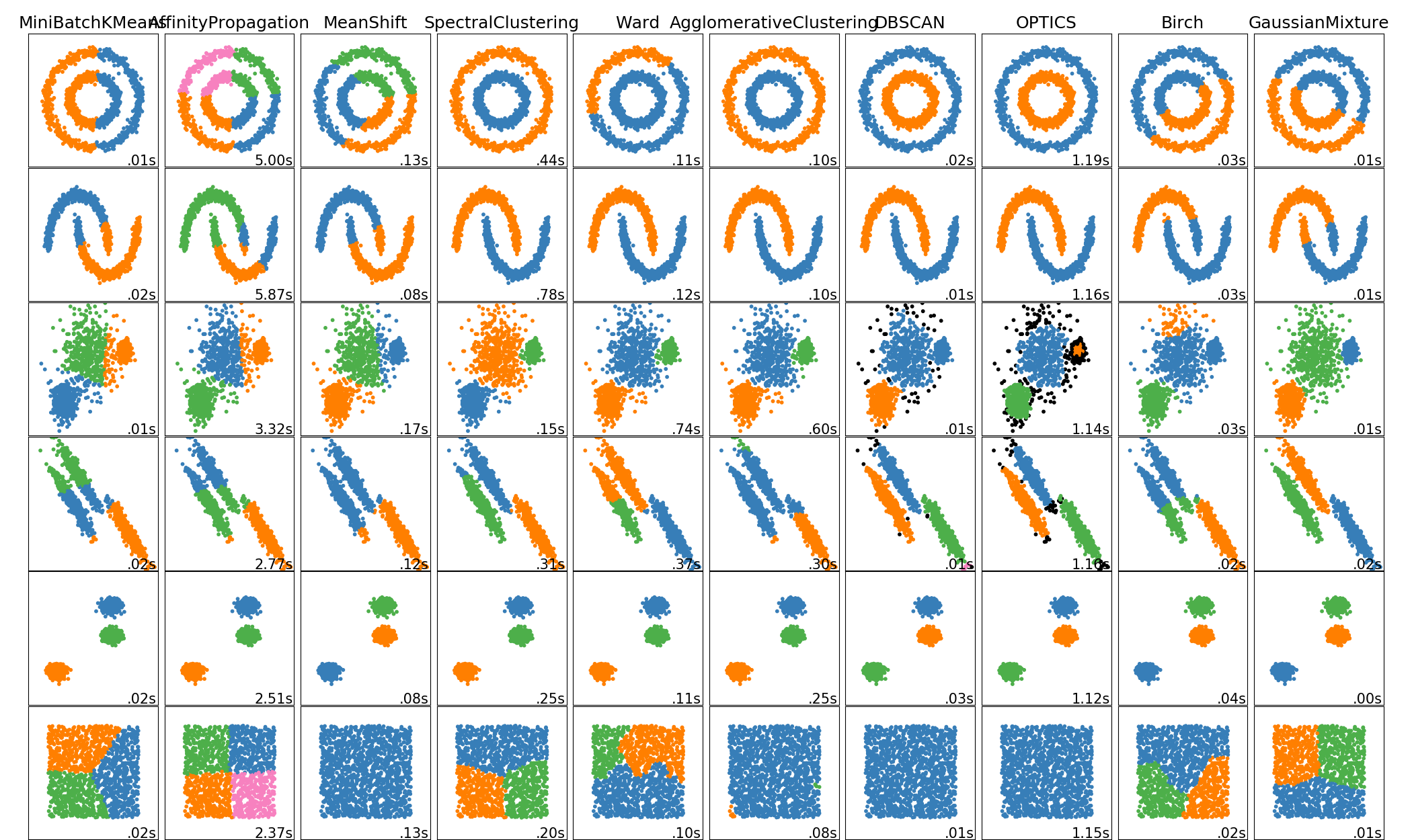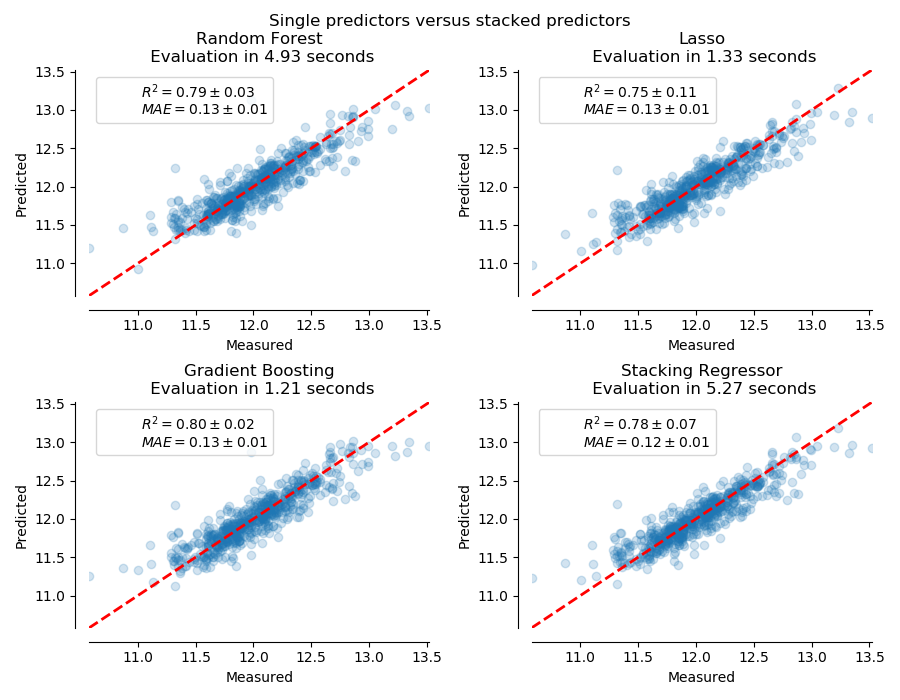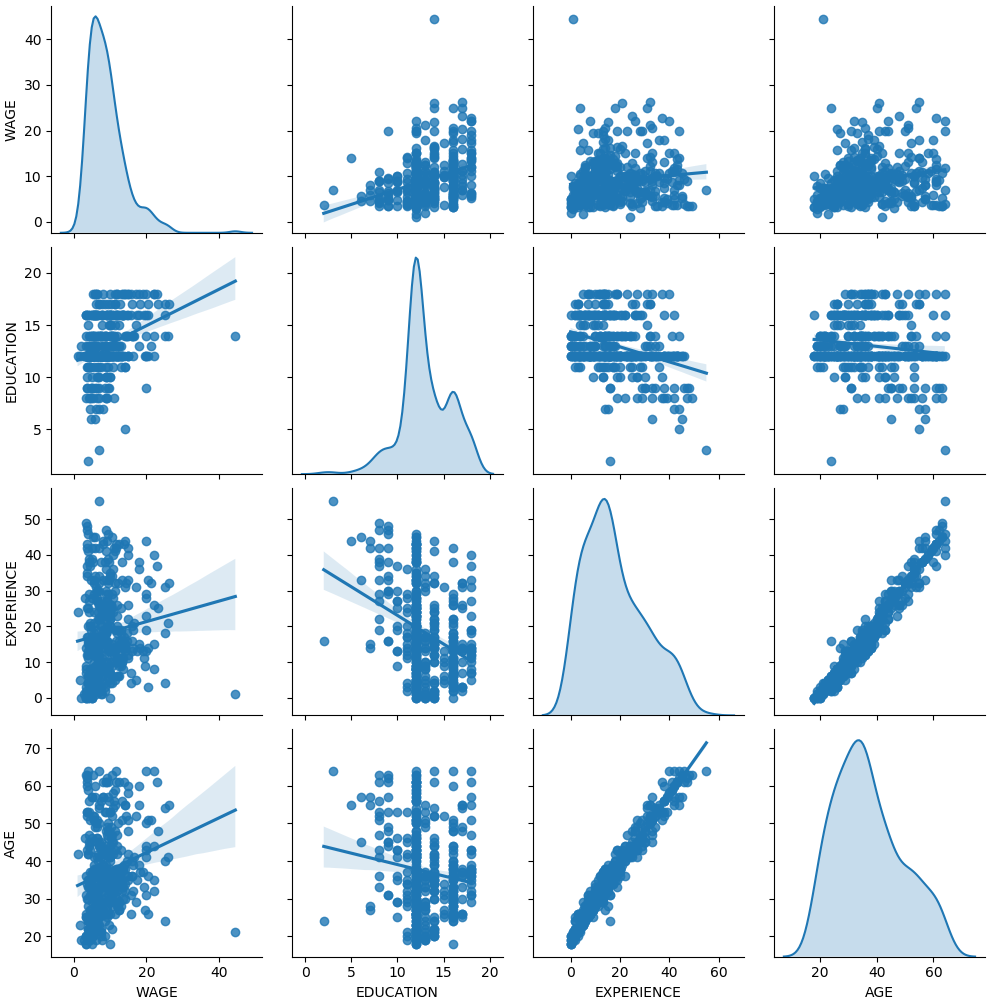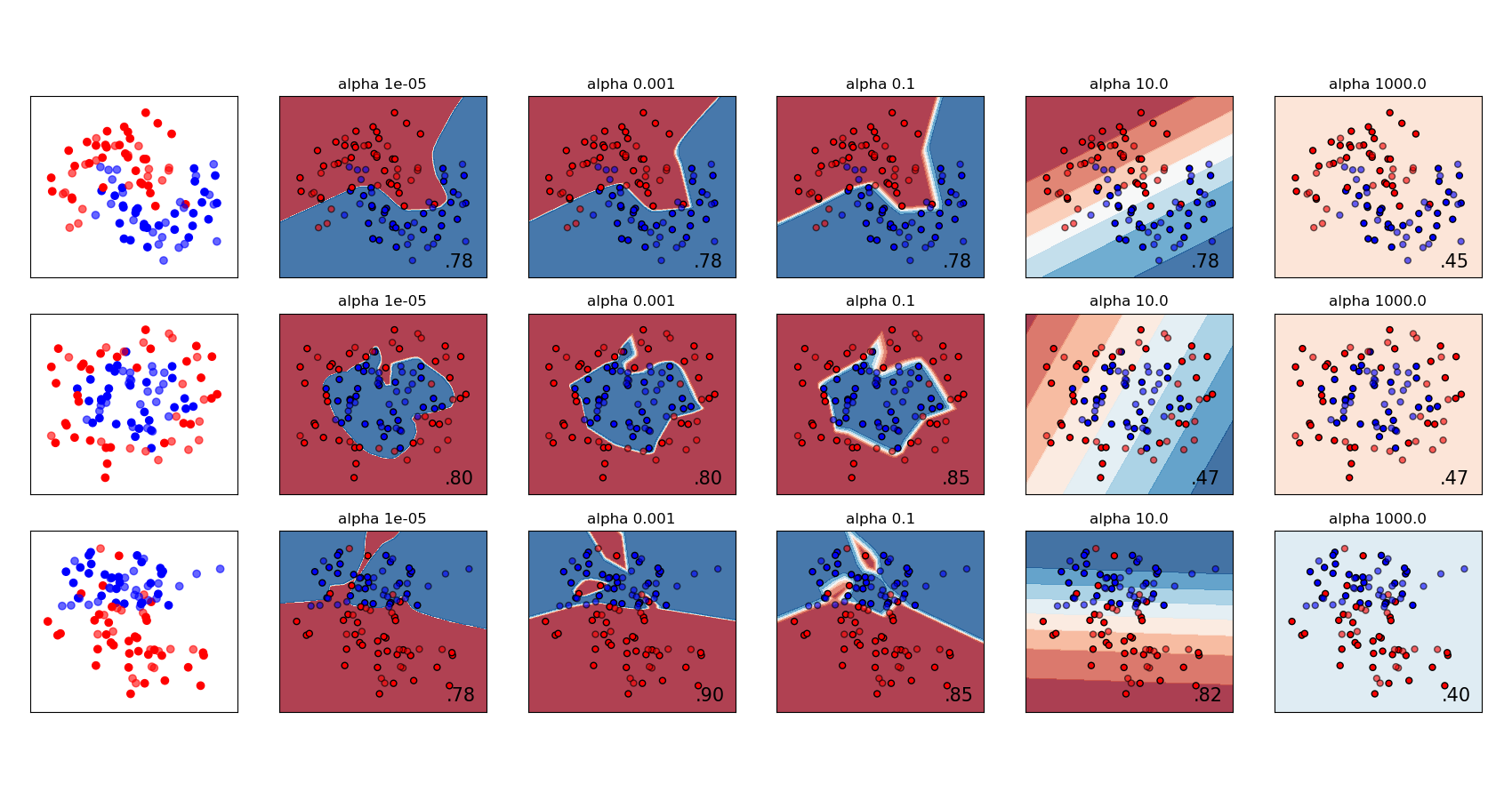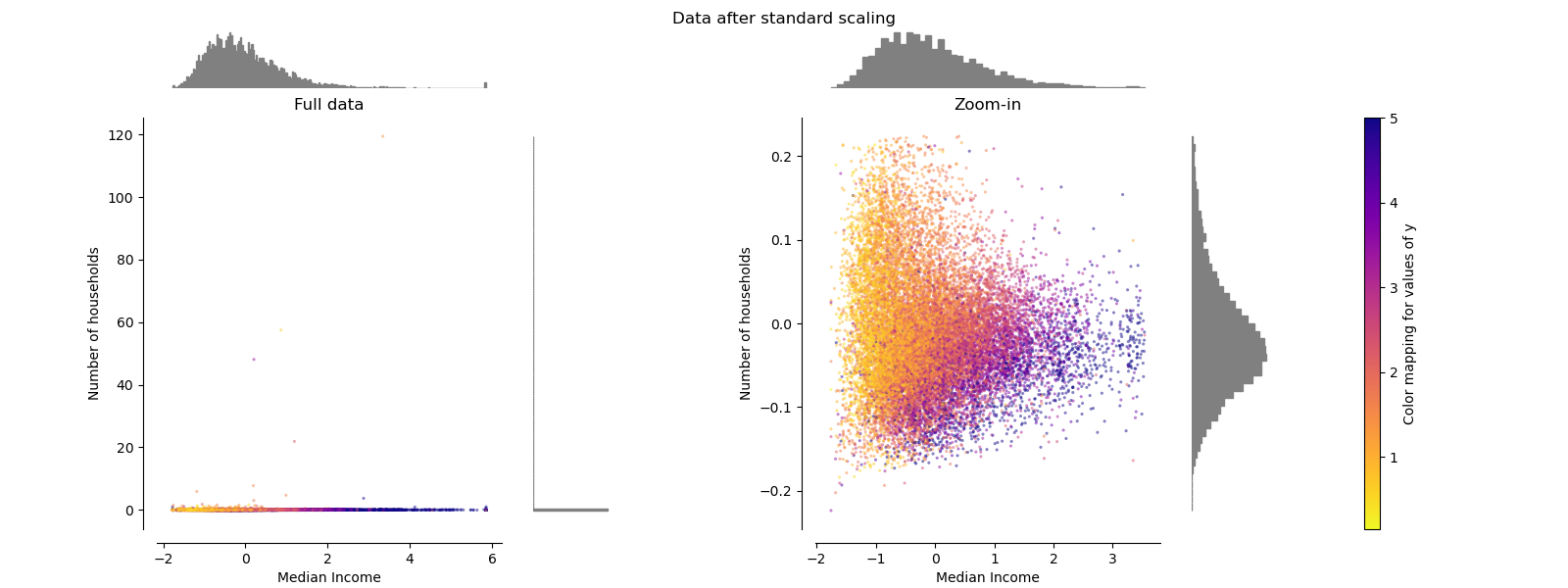# sklearn.preprocessing.StandardScaler¶

class sklearn.preprocessing.StandardScaler(*, copy=True, with_mean=True, with_std=True)

[源码]

z = (x - u) / s

copy boolean, optional, default True

with_mean boolean, True by default

with_std boolean, True by default

scale_ ndarray or None, shape (n_features,)

0.17版中的新功能：scale
mean_ ndarray or None, shape (n_features,)

var_ ndarray or None, shape (n_features,)

n_samples_seen_ int or array, shape (n_features,)

scale

sklearn.decomposition.PCA

NaN被视为缺失值：忽略适合度，并保持变换值。

>>> from sklearn.preprocessing import StandardScaler>>> data = [[0, 0], [0, 0], [1, 1], [1, 1]]>>> scaler = StandardScaler()>>> print(scaler.fit(data))StandardScaler()>>> print(scaler.mean_)[0.5 0.5]>>> print(scaler.transform(data))[[-1. -1.] [-1. -1.] [ 1.  1.] [ 1.  1.]]>>> print(scaler.transform([[2, 2]]))[[3. 3.]]

fit(X[, y]) 计算平均值和标准差，以用于以后的标度。
fit_transform(X[, y]) 拟合数据，然后对其进行转换。
get_params([deep]) 获取此估计量的参数。
inverse_transform(X[, copy]) 将数据按比例缩小到原始表示形式
partial_fit(X[, y]) 在线计算X上的均值和标准差，以便以后缩放。
set_params(**params) 设置此估算器的参数。
transform(X[, copy]) 通过居中和缩放执行标准化
__init__(*, copy=True, with_mean=True, with_std=True)

[源码]

fit(X, y=None)

[源码]

X {array-like, sparse matrix}, shape [n_samples, n_features]

y None

fit_transform(X, y=None, **fit_params)

[源码]

X {array-like, sparse matrix, dataframe} of shape (n_samples, n_features)
y ndarray of shape (n_samples,), default=None

**fit_params dict

X_new ndarray array of shape (n_samples, n_features_new)

get_params(deep=True)

[源码]

deep bool, default=True

params mapping of string to any

inverse_transform(X, copy=None)

[源码]

X array-like, shape [n_samples, n_features]

copy bool, optional (default: None)

X_tr array-like, shape [n_samples, n_features]

partial_fit(X, y=None)

[源码]

Chan，Tony F.，Gene H.Golub和Randall J.LeVeque的公式1.5a，b中给出了增量均值和std的算法。“计算样本方差的算法：分析和建议。”美国统计学家37.3（1983）：242-247：

X {array-like, sparse matrix}, shape [n_samples, n_features]

y None

self object

set_params(**params)

[源码]

**params dict

self object

transform(X, copy=None)

[源码]

X array-like, shape [n_samples, n_features]

X_out bool, optional (default: None)

## sklearn.preprocessing.StandardScaler使用示例¶